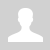Python. Module Random
 code { font-family: Consolas,"courier new"; background: #f4f4f4; border: 1px solid #ddd; border-left: 3px solid #f36d33; color: #666; page-break-inside: avoid; font-size: 15px; line-height: 1.6; margin-bottom: 1.6em; max-width: 100%; overflow: auto; padding: 1em 1.5em; display: block; word-wrap: break-word; } 12.6.1.1 use the functions of the library Random to get a pseudo-random number. 12.6.1.2 determine the function of the library Random for the specified task. 12.6.1.3 identify code snippets that use random sequence generation. Random library This module is designed to work with pseudo-random sequences. Such sequences are important in mathematical modeling, cryptography, and in various games. Let's see the structure of the module. ```import random print(dir(random)) # [... 'choice', 'choices', ... 'randint', 'random', 'randrange', 'sample', ... 'shuffle'...]``` How to import libraries/functions? ```import random # imports all the functions in the library print(random.randint(0,10)) # choses one random number between 0 and 10 (including both) and prints it.``` ```from random import randint # imports only randint function from random library print(randint(0,10)) # choses one random number between 0 and 10(including both) and prints it.``` ```import random as rand # random library can be renamed with this way print(rand.randint(0,10)) # choses one random number between 0 and 10 (including both) and prints it.```Random function Returns a random value between 0 and 1 (0 is inclusive and 1 is exclusive) - [0, 1) ```import random print(random.random()) #0.1149786475411152 print(int(random.random()*100)) #43```Randint/randrange functions Randint: Returns random integer in range [a, b], including both endpoints. ```import random print(random.randint(0,10)) # 10``` Randrange: Chooses a random item from range(start, stop[, step]). It includes the start but not the stop. ```print(random.randrange(0,100, 5))    # 0 print(random.randrange(0,100, 5))    # 10 print(random.randrange(0,100, 5))    # 65``` Choice function Note: use help(random.choice) to get help about choice function after import random statement. ```import random mylist = ["Uralsk", "Kostanay", "Nur-Sultan", "Almaty"] # list of cities print(random.choice(mylist)) # chooses and prints a random city from mylist # Nur-Sultan``` Sample function Chooses k unique random elements from a population sequence or set. Returns a new list containing elements from the population while leaving the original population unchanged. ```import random print(random.sample(mylist, 2)) #['Nur-Sultan', 'Uralsk’] print(random.sample(mylist, 60)) #ValueError: Sample larger than population or is negative``` Choices function Return a k-sized list of population elements chosen with replacement. If the relative weights are not specified, the selections are made with equal probability. ```print(random.choices(mylist, weights=[5,1,5,1], k=5)) #['Nur-Sultan', 'Uralsk', 'Uralsk', 'Nur-Sultan', 'Uralsk'] print(random.choices(mylist, weights=[5,1,5,1], k=5)) #['Nur-Sultan', 'Uralsk', 'Uralsk', 'Uralsk', 'Nur-Sultan'] print(random.choices(mylist, weights=[5,1,5,1], k=5)) #['Uralsk', 'Kostanay', 'Nur-Sultan', 'Nur-Sultan', 'Kostanay']``` Shuffle function Shuffles a given list. ```import random mylist = ['Uralsk', 'Kostanay', 'Nur-Sultan', 'Almaty’] print(mylist) # ['Uralsk', 'Kostanay', 'Nur-Sultan', 'Almaty’] random.shuffle(mylist) print(mylist) # ['Almaty', 'Uralsk', 'Kostanay', 'Nur-Sultan']``` Questions: 1. Name four functions of the Random module. 2. Explain how to use each function. 3. Give examples of real-life tasks where we can apply random functions. Exercises: Ex.1 Tasks: 1. Output random integer from 11 to 20. 2. Create a list of types of applications and output random choice. 3. Write a program to implement "Sportloto" 5 from 36. Output five random numbers in ascending order. 4. Write a program that simulates tossing coins. The number of attempts is entered from the keyboard. The program should display the results of the rolls: heads or tails. 5. A program has been written that determines the "Secret Santa" for all students in the class. Note: a student cannot be a "Secret Santa" for himself. 6. Create the program “Magic ball”. .u-star-rating-13 { list-style:none; margin:0px; padding:0px; width:65px; height:13px; position:relative; background: url('/.s/t/2001/rating13.png') top left repeat-x } .u-star-rating-13 li{ padding:0px; margin:0px; float:left } .u-star-rating-13 li a { display:block;width:13px;height: 13px;line-height:13px;text-decoration:none;text-indent:-9000px;z-index:20;position:absolute;padding: 0px;overflow:hidden } .u-star-rating-13 li a:hover { background: url('/.s/t/2001/rating13.png') left center;z-index:2;left:0px;border:none } .u-star-rating-13 a.u-one-star { left:0px } .u-star-rating-13 a.u-one-star:hover { width:13px } .u-star-rating-13 a.u-two-stars { left:13px } .u-star-rating-13 a.u-two-stars:hover { width:26px } .u-star-rating-13 a.u-three-stars { left:26px } .u-star-rating-13 a.u-three-stars:hover { width:39px } .u-star-rating-13 a.u-four-stars { left:39px } .u-star-rating-13 a.u-four-stars:hover { width:52px } .u-star-rating-13 a.u-five-stars { left:52px } .u-star-rating-13 a.u-five-stars:hover { width:65px } .u-star-rating-13 li.u-current-rating { top:0 !important; left:0 !important;margin:0 !important;padding:0 !important;outline:none;background: url('/.s/t/2001/rating13.png') left bottom;position: absolute;height:13px !important;line-height:13px !important;display:block;text-indent:-9000px;z-index:1 } Категория: Programming languages | Добавил: bzfar77 (03.09.2022) Просмотров: 404 | Теги: Random, sample, randint, Choice, shuffle, Python, Choices | Рейтинг: 5.0/1
 Всего комментариев: 0